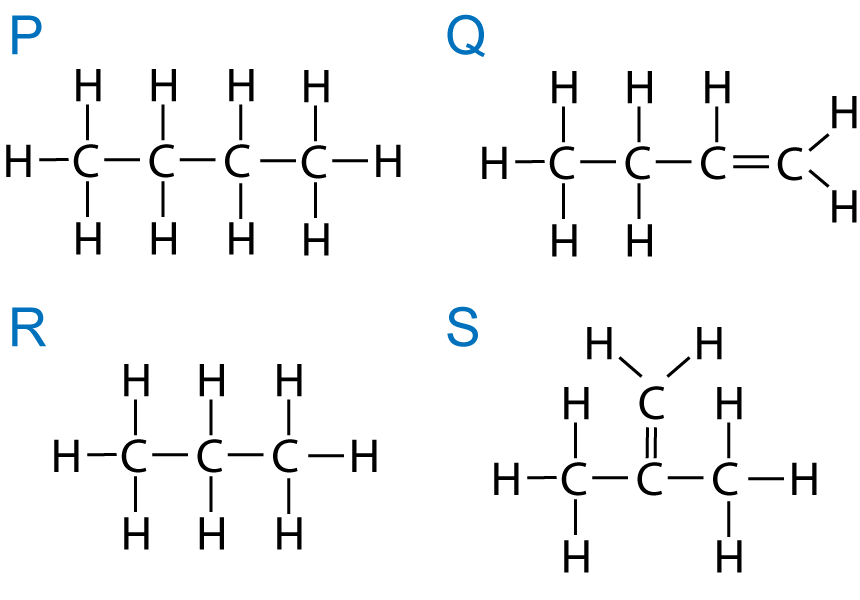Chemistry µGCSE:

Further Hydrocarbons

10 quick questions - for iGCSE

 10 minutes maximum! (can you do it in 5?) Q1-3:Under certain conditions ethane will react slowly with bromine. 1. The conditions required for this reaction are … A.   a temperature of 300oC B.   a catalyst of alumina or silica C.   sunlight or u.v. light D.   a pressure of 60atm ? A B C D 2. The correct equation for the reaction between ethane and bromine is …… A.  C2H6 + Br2  →  C2H5Br + HBr B.  C2H6 + Br2  →  C2H4Br2 + H2 C.  C2H6 + Br2  →  C2H6Br2  D.  C2H4 + Br2  →  C2H4Br2 ? A B C D 3.The reaction between ethane and bromine is a/an ? addition substitution displacement cracking reaction. 4. Isomers are molecules with the … A.   same general formula but a different structural formula B.   same molecular formula but a different structural formula C.   same general formula but a different molecular formula D.   same molecular formula but a different functional group ? A B C D 5. Hexane has the structure:Which one of the following is an isomer of hexane?? A B C D 6. Which of the following structures, X, Y and Z are isomers of C4H8 ?A.   X only B.   X and Y only C.   X and Z only D.   X, Y and Z ? A B C D Q7-10 refer to the following compounds, P, Q, R and S:7. Which compounds are isomers? A.   P and Q B.   P and R C.   R and S D.   Q and S ? A B C D 8. Which compound(s) will decolourise bromine water immediately? A.   P and Q B.   P and R C.   R and S D.   Q and S ? A B C D 9. Which compound(s) can form addition polymers? A.   P and Q B.   P and R C.   R and S D.   Q and S ? A B C D 10. Which compounds are different members of the same homologous series? A.   P and Q B.   P and R C.   R and S D.   Q and S ? A B C D